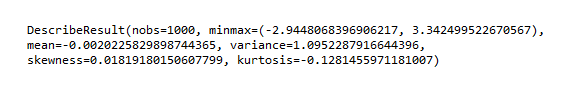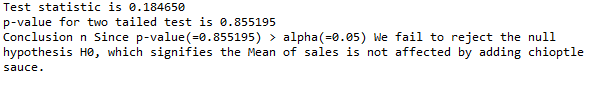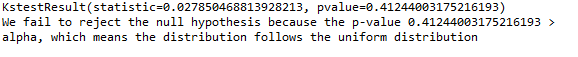Open in App
Not now

# SciPy – Statistical Significance Tests

• Last Updated : 29 Mar, 2022

In probability and statistics, we tend to compute a lot of different numbers, the job doesn’t end there, it is highly essential to statistically interpret the numbers to understand the significance of that number to the particular problem at hand. In statistics, there are few techniques to assess the significance of these numbers. In the article let us discuss a few of the Statistical Significance tests supported by the Python package.

Some of the prominent and widely used statistical significance tests are as follows,’

## Descriptive statistical terms

Mean, median, mode, variance, and standard deviation are popularly called measures of centrality and spread. It has to be the first step in any statistical data analysis.

Skewness and kurtosis are two tests that test for normality. If Skwness is 0 and kurtosis is 3, then it signifies that the distribution of data is perfectly normal (symmetric). If we conclude the distribution to be normal, we can infer a lot of other parameters about the distribution.

If the value of skewness is negative, then the data is left-skewed and if the value is positive then the data is right-skewed. Similarly, positive kurtosis indicates heavy-tailed distribution and vice versa

## Python3

 `import` `numpy as np` `from` `scipy.stats ``import` `describe`   `v ``=` `np.random.normal(size``=``100``)` `result ``=` `describe(v)`   `print``(result)`

Output:## Hypothesis tests

Hypothesis testing is a statistical test that uses data from a sample to draw conclusions about a population parameter. Hypothesis testing is conducted by defining an experiment and testing for the statistical significance of the assumption.

Null Hypothesis Ho: Assumes no statistical relationship and significance exists in a set of given single observed variable.

Alternate Hypothesis H1: Assumes there is a statistical relationship of a single observed variable.

The parameters of hypothesis tests include p-values and alpha values (specifying the significance level). The p-value measures the probability of getting a more extreme value than the one you got from the experiment. Alpha, the significance level, is the probability that you will make the mistake of rejecting the null hypothesis when in fact it is true. If p-value <= alpha we reject the null hypothesis and say that the data is statistically significant. else, we accept the null hypothesis.

Hypothesis testing is of two types:

• One-tailed test
• Two-tailed test

### One-Tailed test

A one-tailed test is a statistical test in which the critical area of a distribution is one-sided so that it is either greater than or less than a certain value, but not both.

### Two-Tailed test

A two-tailed test is a method in which the critical area of a distribution is two-sided and tests whether a sample is greater than or less than a certain range of values.

Now, let us use this concept of hypothesis testing to demonstrate t-test.

### T-Test

The T-test is used to determine if there is a significant difference between the means of two groups in the study. This test assumes that the data follows t – distribution, which is a slight variation of the normal distribution.

Example: Let us try and test the following assumption. Say, a company named ‘X’ wishes to test whether the Mean sales is affected by adding a chipotle sauce in its best-selling dish. Now, let’s frame a hypothesis for this problem.

Null Hypothesis Ho:  Mean of sales is not affected because of adding the chipotle sauce μ1 = μ2

Alternate Hypothesis H1: Mean of sales is affected because of adding the chipotle sauce μ1 != μ2

The confidence interval is chosen as 95% for this problem, so the alpha value = 0.05. We know that if p <= alpha rejects the null hypothesis. We make use of the ttest_ind() function in the scipy to conduct the t-test as shown below.

## Python3

 `from` `scipy ``import` `stats`   `sales_with_chipotle ``=` `[``13.4``, ``10.9``, ``11.2``, ``11.8``,` `                       ``14``, ``15.3``, ``14.2``, ``12.6``, ` `                       ``17``, ``16.2``, ``16.5``, ``15.7``]`   `sales_without_chipotle ``=` `[``12``, ``11.7``, ``10.7``, ``11.2``,` `                          ``14.8``, ``14.4``, ``13.9``, ``13.7``,` `                          ``16.9``, ``16``, ``15.6``, ``16``]`   `t_value, p_value ``=` `stats.ttest_ind(sales_with_chipotle,` `                                   ``sales_without_chipotle)`   `print``(``'Test statistic is %f'` `%` `float``(``"{:.6f}"``.``format``(t_value)))` `print``(``'p-value for two tailed test is %f'` `%` `p_value)`   `alpha ``=` `0.05` `if` `p_value <``=` `alpha:`   `    ``print``(``'Conclusion'``, ``'n'``, ``'Since p-value(=%f)'` `%` `p_value, ` `          ``'<'``, ``'alpha(=%.2f)'` `%` `alpha, ` `          ``'''We reject the null hypothesis H0. \` `          ``So we conclude that the \` `          ``Mean sales is affected by \` `          ``adding chipotle sauce to the best\` `          ``selling ingredient i.e., μ1 = μ2 at \` `          ``%.2f level of significance.'''` `%` `alpha)`   `else``:`   `    ``print``(``'Conclusion'``, ``'n'``, ``'Since p-value(=%f)'` `%` `p_value,` `          ``'>'``, ``'alpha(=%.2f)'` `%` `alpha,` `          ``'We fail to reject the null \` `          ``hypothesis H0, which signifies \` `          ``the Mean of sales ``is` `not` `affected\` `          ``by adding chipotle sauce.')`

Output:## Kolmogorov Smirnoff test

The Kolmogorov-Smirnov test is used to test the null hypothesis that a set of data comes from a similar distribution. KS test Performs the one-sample or two-sample test for goodness of fit. The one-sample test compares the underlying distribution F(x) of a sample against a given distribution G(x). The two-sample test compares the underlying distributions of two independent samples. Both tests are valid only for continuous distributions.

Example: Here we have generated a sample of 1000 values that follows a uniform distribution using a random function.  Then we import the kstest() function to test whether the random function correctly generates the uniform distribution. Let us choose the alpha value as 0.05

Null Hypothesis Ho: The distribution follows a uniform distribution

Alternate Hypothesis H1: The distribution doesn’t follow a uniform distribution.

The p-value is calculated using test statistics returned by kstest function. If pvalue> alpha, we fail to reject the null hypothesis else accept the alternate hypothesis

## Python3

 `import` `numpy as np` `from` `scipy.stats ``import` `kstest`   `v ``=` `np.random.uniform(size``=``1000``)`   `res ``=` `kstest(v, ``'uniform'``)`   `print``(res)` `      `  `alpha ``=` `0.05`   `if` `res[``1``] > alpha:` `    ``print``('We fail to reject the null hypothesis because \` `    ``the p``-``value {res[``1``]} > alpha, which means the\` `    ``distribution follows the uniform distribution')`   `else``:` `    ``print``('We  reject the null hypothesis because\` `    ``the p``-``value {res[``1``]} < alpha, which means the\` `    ``distribution doesn"t follows the uniform distribution')`

Output:My Personal Notes arrow_drop_up
Related Articles